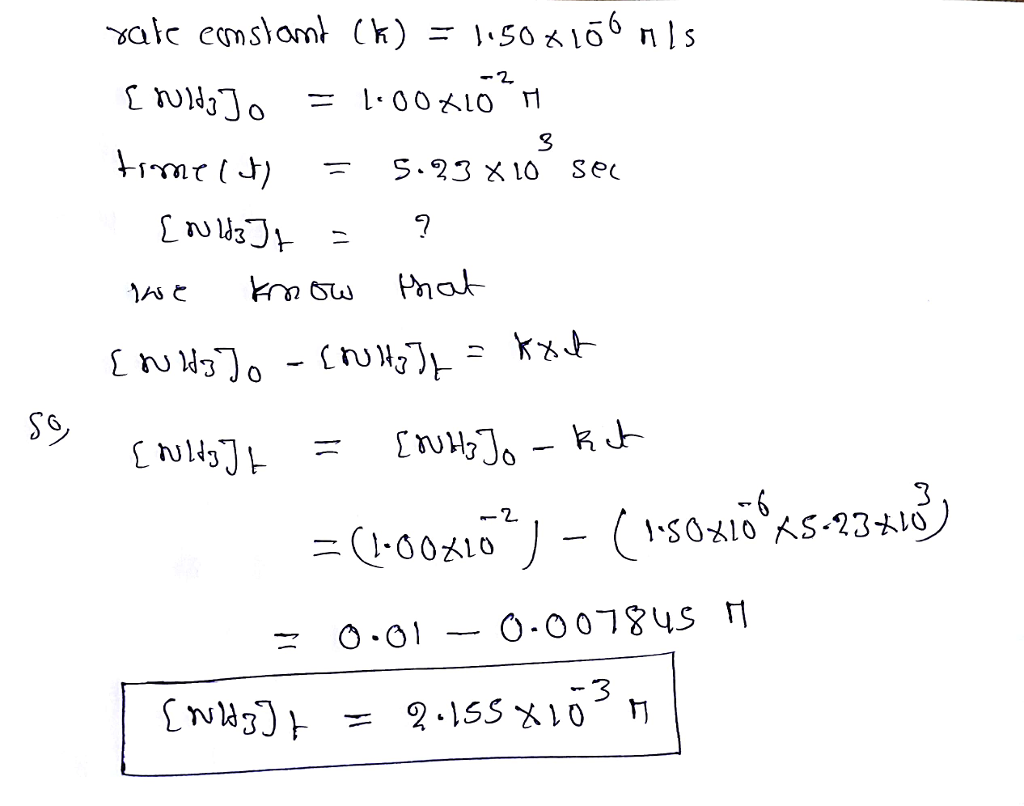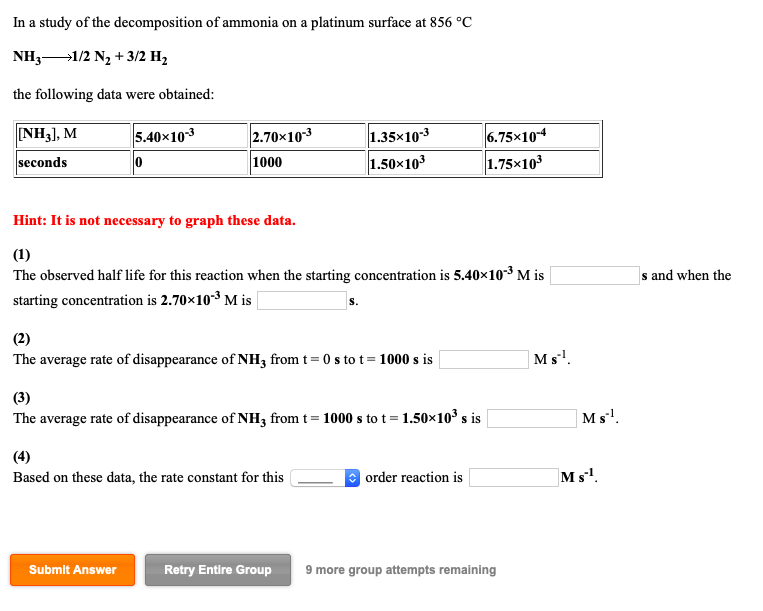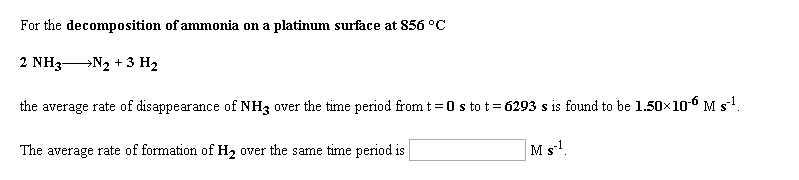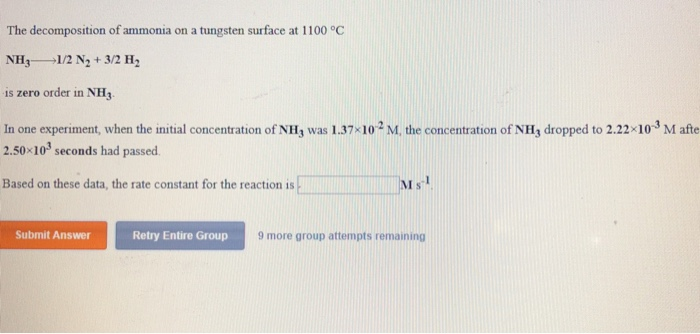Question

# The decomposition of ammonia on a platinum surface at 856 °C NH3-------> 1/2 N2 + 3/2...

The decomposition of ammonia on a platinum surface at 856 °C

NH3-------> 1/2 N2 + 3/2 H2

is zero order in NH3 with a rate constant of 1.50×10-6 M s-1.

If the initial concentration of NH3 is 1.00×10-2 M, the concentration of NH3 will be _______________ M after 5.23×103 seconds have passed.#### Earn Coins

Coins can be redeemed for fabulous gifts.

Similar Homework Help Questions
• ### The decomposition of ammonia on a platinum surface at 856 °C NH31/2 N2 + 3/2 H2...

The decomposition of ammonia on a platinum surface at 856 °C NH31/2 N2 + 3/2 H2 is zero order in NH3 with a rate constant of 1.50×10-6 M s-1. If the initial concentration of NH3 is 7.92×10-3 M, the concentration of NH3 will be M after 4.29×103 seconds have passed.

• ### For the decomposition of ammonia on a platinum surface at 856 °C 2 NH3-N2 + 3...For the decomposition of ammonia on a platinum surface at 856 °C 2 NH3-N2 + 3 H2 the average rate of disappearance of NH; over the time period from t = 0 stot - 852 s is found to be 1.50~10 Ms!. The average rate of formation of H, over the same time period is Ms! Submit Answer Try Another Version 10 item attempts remaining Visited Prev

• ### In a study of the decomposition of ammonia on a platinum surface at 856 °C NH31/2...In a study of the decomposition of ammonia on a platinum surface at 856 °C NH31/2 N2+3/2 H2 the following data were obtained NH3], M 1.35x10-3 5.40x103 2.70x103 6.75x104 1.50x103 1.75x103 seconds 1000 Hint: It is not necessary to graph these data. (1) The observed half life for this reaction when the starting concentration is 5.40x103 M is s and when the starting concentration is 2.70x103 M is (2) Ms The average rate of disappearance of NH3 from t 0s...

• ### For the decomposition of ammonia on a platinum surface at 856 °C 2 NH3-N2 + 3...For the decomposition of ammonia on a platinum surface at 856 °C 2 NH3-N2 + 3 H2 the average rate of disappearance of NH3 over the time period from t=0 s to t = 6293 s is found to be 1.50X100 Ms? The average rate of formation of H, over the same time period is Ms. For the decomposition of hydrogen peroxide in dilute sodium hydroxide at 20 °C 2 H2O2(aq) +2 H2O(1) + O2(g) the average rate of disappearance...

• ### The decomposition of ammonia on a tungsten surface at 1100 °C NH1/2 N +3/2 H is...The decomposition of ammonia on a tungsten surface at 1100 °C NH1/2 N +3/2 H is zero order in NH3 In one experiment, when the initial concentration of NH, was 1.37-102 M, the concentration of NHj dropped to 2.22 103 M afte 2.50 103 seconds had passed Based on these data, the rate constant for the reaction is M s Submit Answer Retry Entire Group 9 more group attempts remaining

• ### In a study of the decomposition of ammonia on a tungsten surface at 1100 °C NH31/2...

In a study of the decomposition of ammonia on a tungsten surface at 1100 °C NH31/2 N2 + 3/2 H2 the following data were obtained: [NH3], M 6.69×10-3 3.35×10-3 1.68×10-3 8.40×10-4 seconds 0 441 661 772 Hint: It is not necessary to graph these data. (1) The observed half life for this reaction when the starting concentration is 6.69×10-3 M is s and when the starting concentration is 3.35×10-3 M is s. (2) The average rate of disappearance of NH3...

• ### 1A. The decomposition of dinitrogen pentoxide in carbon tetrachloride solution at 30 °C N2O5 2 NO2...1A. The decomposition of dinitrogen pentoxide in carbon tetrachloride solution at 30 °C N2O5 2 NO2 + ½ O2 is first order in N2O5 with a rate constant of 4.10×10-3 min-1. If the initial concentration of N2O5 is 0.510 M, the concentration of N2O5 will be M after 402 min h 1B. The gas phase decomposition of phosphine at 120 °C PH3(g)1/4 P4(g) + 3/2 H2(g) is first order in PH3 with a rate constant of 1.80×10-2 s-1. If the...

• ### NH3 →1/2 N2 + 3/2 H2 the following data were obtained:

NH3 →1/2 N2 + 3/2 H2the following data were obtained:[NH3], M1.67×10-38.35×10-44.18×10-42.09×10-4seconds010001.50×1031.75×103Hint: It is not necessary to graph these data.(1)The observed half life for this reaction when the starting concentration is 1.67×10-3 M iss and when the starting concentration is8.35×10-4 M is ________s.(2)The average rate of disappearance ofNH3 from t = 0 s to t = 1000 s is ______Ms-1.(3)The average rate of disappearance ofNH3 from t = 1000s to t = 1.50×103s is _______Ms-1.(4)Based on these data, the rate constant...

• ### The decomposition of nitrous oxide at 565 °C N2O(g)----> N2(g) + ½ O2(g) is second order...

The decomposition of nitrous oxide at 565 °C N2O(g)----> N2(g) + ½ O2(g) is second order in N2O. In one experiment, when the initial concentration of N2O was 0.790 M, the concentration of N2O dropped to 0.103 M after 5.21×10^3 seconds had passed. Based on these data, the rate constant for the reaction is _____________ M-1 s-1.

• ### The decomposition of ammonia gas (NH3) leads to the production of N2 gas and H2 gas....

The decomposition of ammonia gas (NH3) leads to the production of N2 gas and H2 gas. A chemist adds 4.0 mol of ammonia gas to a 2.0 L sealed, rigid container and heats it. It has been found that at equilibrium the number of moles of ammonia gas is 2.0 in the same 2.0 L container. Determine the equilibrium concentration of N2 and H2 gas,